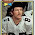## Friday, July 12, 2019

### 49 127 | 4.9 magnitude earthquake hits Ridgecrest, July 12, 2019

https://www.cnn.com/2019/07/12/us/ridgecrest-california-earthquake/index.html

More than 4,700 quakes since the July 4 quake, eh?

That follows the paragraph about "11 miles Northeast of Ridgecrrest, the USGS said.  It unleashed 11 times the amount of energy"...

This latest quake, a 4.9, comes July 12, or 12/7, like 127.

Scottish Rite of Freemasonry = 127
Scottish = 49; America = 49

*There was also a 4.6 quake in thee Seattle area today.

1.07 + 12 + 20 + 19 = 58
Matches:
08 + 11 + 20 + 19 = 58

"Agenda" = 58, 58 <<< Gematriot
"D.O.D." = 58
"Eight Eleven" = 58
"Hidden Agenda" = 58
"Fake Earthquake" = 58

4.9 Magnitude?

"Planned Chaos" = 49
"Future Event" = 49
"Revelation" = 49
"Sign" = 49
"Key" = 49
"End" = 49

"Forty Nine" = 126
"Scripted Agenda" = 126

"July Twelfth Message" = 69
"One Month One Day" = 69 <<< What Day is That?
"Count Thirty Days" = 69
"Hundred Eighteen" = 69
"Thirtieth Day" = 69
"Message" = 69
"Event" = 69
"Magic" = 69

Todays Date is 721 or 127

"M.A.G.A. Symbols" = 127 & 721 <<<<<<<<<<<<<<
"Symbolism" = 721

Notice the Post Time of 10:42 Am, like 142

"Black Operation" = 142
"Coming Mega-Quake" = 142

42nd Minute Leaves 18 Minutes: 6 + 6 + 6

"Prophecy" = 666
"Orders to Proceed" = 666
"Earthquake Machines" = 666
"Ritualistic Kabbalah" = 666
"Scripted Agenda Signals" = 666
"Gematria Language Codes" = 666
"Mathematical Perfection" = 666 <<< 36th Triangular

6 * 6 * 6 = 216

"Combined Disasters" = 612 <<< Illuminati Card Game

1.07 + 12 + 20 + 19 = 58

"Ridgecrest CA" = 58, 112 <<< Old Emergency Dialing Code?
"Eight Eleven" = 58, 112

2.3.Jake nice work